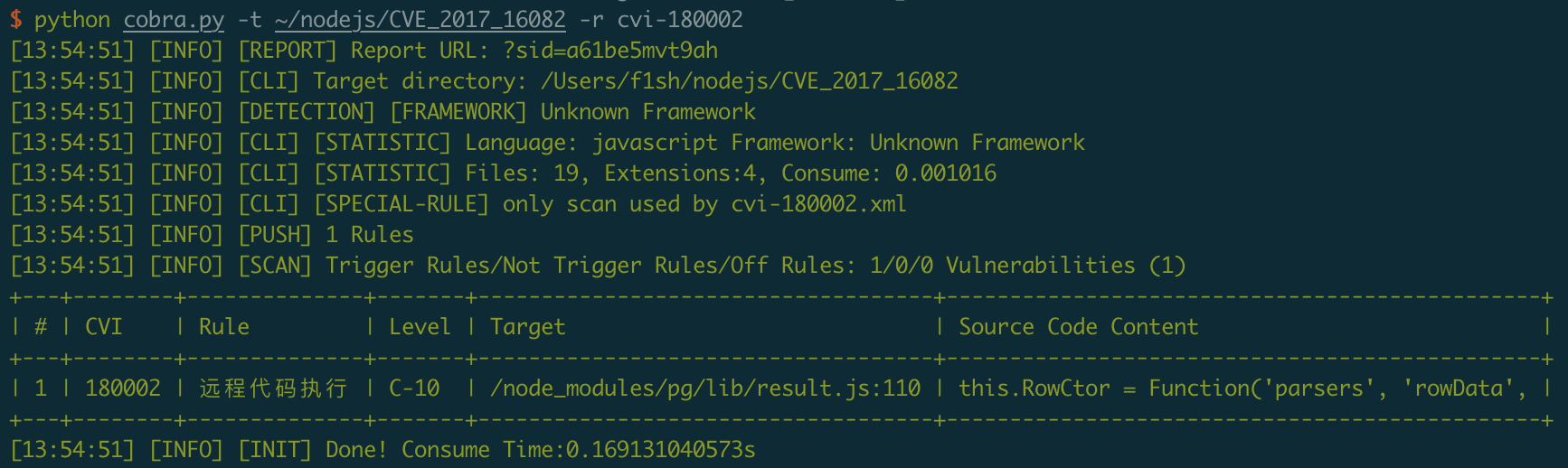# 给 Cobra 增加 Node.js 源代码扫描规则

Cobra是一款源代码安全审计工具，支持检测多种开发语言源代码中的大部分显著的安全问题和漏洞。

``````const app = new Koa()
const client = new Client({
user: "f1sh",
database: "postgres",
host: "127.0.0.1",
port: 5432
})
client.connect()

app.use(async ctx => {
ctx.response.type = 'html'

let id = ctx.request.query.id || 1
let sql = `SELECT * FROM "user" WHERE "id" = \${id}`
const res = await client.query(sql)

ctx.body = `<html>
<body>
<table>
<tr><th>id</th><td>\${res.rows.id}</td></tr>
<tr><th>name</th><td>\${res.rows.name}</td></tr>
<tr><th>score</th><td>\${res.rows.score}</td></tr>
</table>
</body>
</html>`
})

app.listen(3000)
``````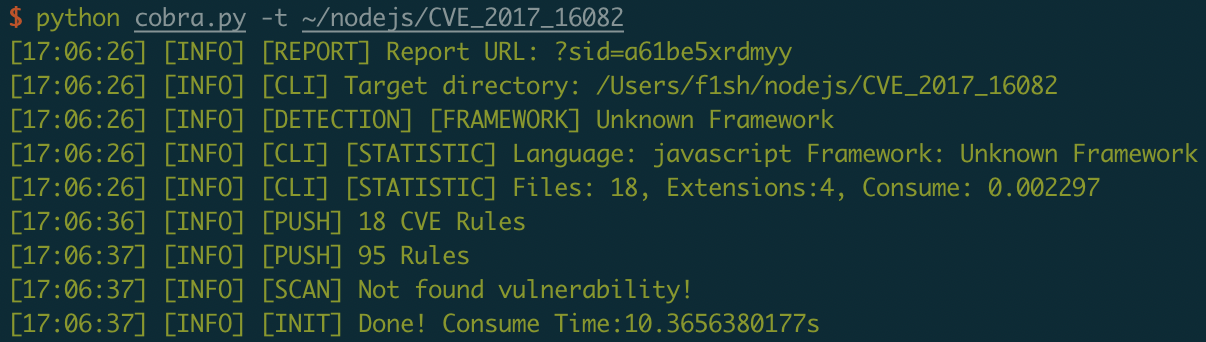``````<?xml version="1.0" encoding="UTF-8"?>

<cobra document="https://github.com/WhaleShark-Team/cobra">
<name value="拼接SQL注入"/>
<language value="javascript"/>
<match mode="regex-only-match"><![CDATA[(['"`]\s*(select|SELECT|insert|INSERT|update|UPDATE|delete|DETELE)[^\w.]+[^;]*?(\\$\{(.+?)\}|['"`]\s*\+\s*[\$a-zA-Z_][\$\w]?))]]></match>
<level value="8"/>
<test>
<case assert="true"><![CDATA[let sql = `SELECT * FROM "user" WHERE "id" = \${id}`]]></case>
<case assert="false"><![CDATA[let sql = 'SELECT * FROM "user" WHERE "id" = 1']]></case>
<case assert="true"><![CDATA[let sql = 'SELECT * FROM "user" WHERE "id" = ' + id]]></case>
<case assert="true"><![CDATA[const result = await req.db.run(`UPDATE notes SET pinned = \${req.body.value}::boolean WHERE id = \${req.params.noteId}`);]]></case>
</test>
<solution>
## 安全风险
拼接SQL语句存在SQL注入风险

## 修复方案
- 已不再使用的功能，直接做下线处理
- 引入安全组件
- 代码层修复:尽量不要拼接SQL语句。如必要，需对用户可控的参数进行严格过滤
</solution>
<status value="on"/>
<author name="f1sh" email="[email protected]"/>
</cobra>
``````

``````['"`]\s*(select|SELECT|insert|INSERT|update|UPDATE|delete|DETELE)
``````

``````[^\w.]+[^;]*?
``````

``````(\\$\{(.+?)\}|['"`]\s*\+\s*[\$a-zA-Z_][\$\w]?)
``````

``````let sql = `SELECT * FROM "user" WHERE "id" = \${id}`
let sql = 'SELECT * FROM "user" WHERE "id" = ' + id
``````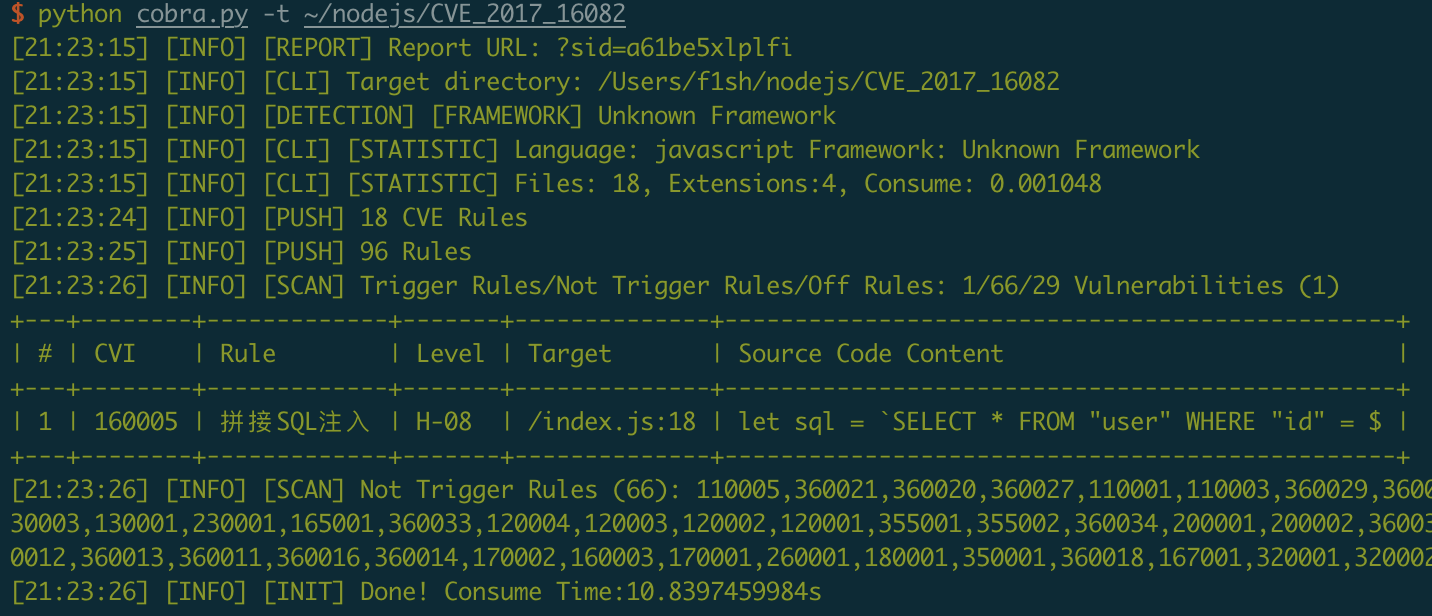``````<?xml version="1.0" encoding="UTF-8"?>

<cobra document="https://github.com/WhaleShark-Team/cobra">
<name value="远程代码执行"/>
<language value="javascript"/>
<match mode="regex-only-match"><![CDATA[([^\w]Function\(.*?([\$\w]+|(['"`].*?(\\$\{(.+?)\}|['"`]\s*\+\s*[\$\w]+).*?))\s*\))]]></match>
<level value="10"/>
<test>
<case assert="true"><![CDATA[this.RowCtor = Function('parsers', 'rowData', ctorBody)]]></case>
<case assert="true"><![CDATA[this.RowCtor = Function('parsers', 'rowData', 'var ' + ctorBody + ';')]]></case>
<case assert="true"><![CDATA[this.RowCtor = Function('parsers', 'rowData', `var \${ctorBody};`)]]></case>
<case assert="false"><![CDATA[var sum = new Function('a', 'b', 'return a + b');]]></case>
</test>
<solution>
## 安全风险
代码注入(Code Injection) - Funtion()
没有对用户输入的参数进行足够的过滤，可能导致攻击者传入的恶意代码执行。
因此危险函数应该尽量避免在开发中使用。

## 修复方案
不要把用户可控的参数带入 Function 的最后一个参数中，或者进行安全的过滤后再带入。
</solution>
<status value="on"/>
<author name="f1sh" email="[email protected]"/>
</cobra>
``````

``````this.RowCtor = Function('parsers', 'rowData', ctorBody)
this.RowCtor = Function('parsers', 'rowData', 'var ' + ctorBody + ';')
this.RowCtor = Function('parsers', 'rowData', `var \${ctorBody};`)
``````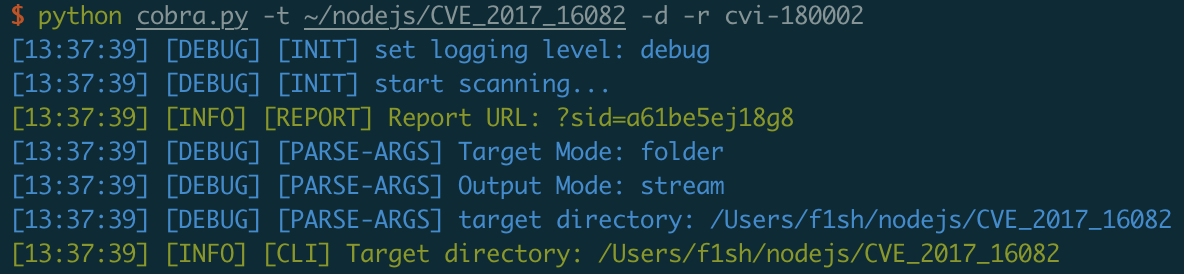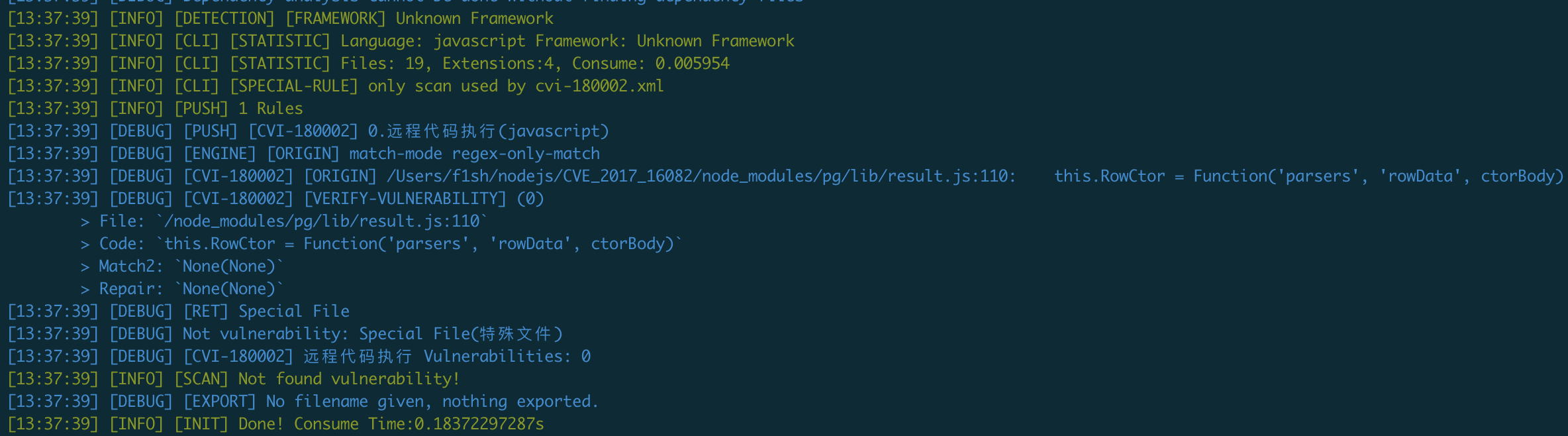``````[13:37:39] [DEBUG] Not vulnerability: Special File(特殊文件)
``````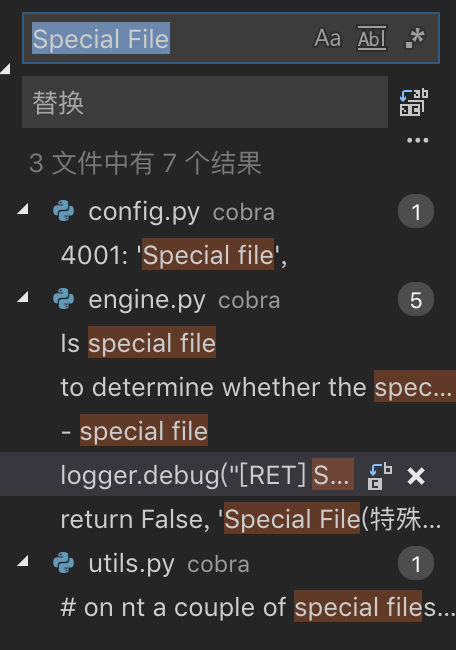``````if self.is_special_file():
logger.debug("[RET] Special File")
return False, 'Special File(特殊文件)'
``````

``````def is_special_file(self):
"""
Is special file
:method: According to the file name to determine whether the special file
:return: boolean
"""
special_paths = [
'/node_modules/',
'/bower_components/',
'.min.js',
'.log',
'.log.',
'nohup.out',
]
for path in special_paths:
if path in self.file_path:
return True
return False
``````